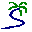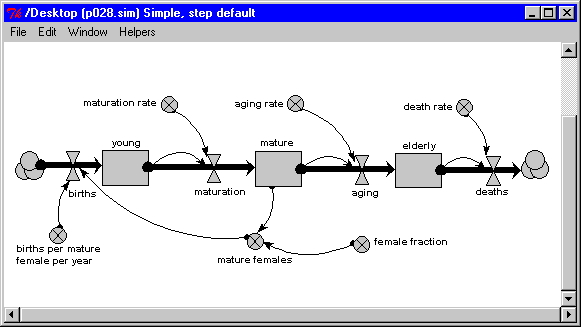# Model entry

Population dynamics: single species: age class: separate compartments - Model catalogue - Simulistics.com

 Search Simulistics Model catalogue Listed by keyword Listed by ID Listed by title Listed by date added

# Population dynamics: single species: age class: separate compartments

Model : popdyn2
Simile version : 3.1+
Keywords : Population dynamics ; Age-class modelling ; Disaggregated population ; Single-species population dynamics ;

## Description

This models the dynamics of a population divided into three age classes: young, mature and elderly. Each age class is represented by a single compartment, whose value is the number of individuals in that class.

The flows between the age classes represent the ageing process: the movement of individuals from one class to the next as the grow older. The flows into the first age class represents reproduction. This depends on the number of mature females, which is a fraction of the number of mature individuals. The flow out of the third age class represents mortality: in this model, we assume that only the old individuals die.

Simple proportional relationships are used for all flows:

• a certain proportion of each class age each year;
• a certain proportion of the females reproduce each year;
• a certain proprtion of the elderly individuals die each year.

The model uses a time step of 1 year.

Source: Andrew Ford (1999): Modelling the Environment, p.28

## Files

### Model file

Click on the icon to download the model file. (You will need Simile to examine and run the model. A free evaluation version is available from the products page.)

Some browsers may attempt to display the model file, rather than open it in Simile; in this case, use the browser back button to return to this page, and use the context menu (invoked by right-clicking on the link) to save the target file to disk.p028.sml

## Diagram## Equations

```Compartments:
elderly
Initial value = 20
Rate of change =  aging - deaths

mature
Initial value = 30
Rate of change =  maturation - aging

young
Initial value = 50
Rate of change =  births - maturation
Flows:
aging = aging_rate*mature
Comments: Number maturing, millions per year

births = births_per_mature_female_per_year*mature_females

deaths = death_rate*elderly

maturation = maturation_rate*young
Comments: Number maturing, millions per year

Variables:
mature females = female_fraction*mature
Comments: Number of mature females, millions

Parameters:
aging rate = 0.1

births per mature female per year = 0.5
Comments: Number of births per female per year

death rate = 0.2

female fraction = 0.5
Comments: Proportion of females in the population

maturation rate = 0.1
Comments: Proportion of young maturing, per year
```

## Results

||

## References

Ford, A (1999) Modeling the Environment.
Island Press. p.28